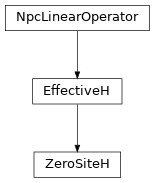# ZeroSiteH¶

Inheritance DiagramMethods

 `ZeroSiteH.__init__`(env, i0) Return the hermitian conjugate of self. Combine the legs of theta, such that it fits to how we combined the legs of self. `ZeroSiteH.from_LP_RP`(LP, RP[, i0]) `ZeroSiteH.matvec`(theta) Apply the effective Hamiltonian to theta. Contract self to a matrix. `ZeroSiteH.update_LP`(env, i[, U]) Equivalent to `env.get_LP(i, store=True)`; optimized for combine. `ZeroSiteH.update_RP`(env, i[, VH]) Equivalent to `env.get_RP(i, store=True)`; optimized for combine.

Class Attributes and Properties

class tenpy.algorithms.mps_common.ZeroSiteH(env, i0)[source]

Bases: `EffectiveH`

Class defining the zero-site effective Hamiltonian for Lanczos.

The effective zero-site Hamiltonian looks like this:

```|        .---    ---.
|        |          |
|       LP----------RP
|        |          |
|        .---    ---.
```

Note that this class has less funcitonality than the `OneSiteH` and `TwoSiteH`.

Parameters
• env (`MPOEnvironment`) – Environment for contraction `<psi|H|psi>`.

• i0 (int) – Site index such that LP is everything strictly left of i0.

length

Number of (MPS) sites the effective hamiltonian covers.

Type

int

acts_on

Labels of the state on which self acts. NB: class attribute. Overwritten by normal attribute, if combine.

Type

list of str

combine, move_right

See above.

Type

bool

LHeff, RHeff

Only set if `combine`, and only one of them depending on `move_right`. If move_right was True, LHeff is set with labels `'(vR*.p0)', 'wR', '(vR.p0*)'` for bra, MPO, ket; otherwise RHeff is set with labels `'(p0*.vL)', 'wL', '(p0, vL*)'`

Type

`Array`

LP, W0, RP

Tensors making up the network of self.

Type

`Array`

matvec(theta)[source]

Apply the effective Hamiltonian to theta.

Parameters

theta (`Array`) – Labels: `vL, vR`.

Returns

Product of theta and the effective Hamiltonian.

Return type

theta `Array`

to_matrix()[source]

Contract self to a matrix.

Return the hermitian conjugate of self.

combine_theta(theta)[source]

Combine the legs of theta, such that it fits to how we combined the legs of self.

Parameters

theta (`Array`) – Wave function to apply the effective Hamiltonian to, with uncombined legs.

Returns

theta – Wave function with labels as given by self.acts_on.

Return type

`Array`

update_LP(env, i, U=None)[source]

Equivalent to `env.get_LP(i, store=True)`; optimized for combine.

Parameters
• env (`MPOEnvironment`) – The same environment as given during class initialization.

• i (int) – We update the part left of site i. Can optimize if i == `i0` and `combine` is True.

• U (None | `Array`) – The tensor on the left-most site self acts on, with combined legs after SVD. Only used if trying to optimize.

update_RP(env, i, VH=None)[source]

Equivalent to `env.get_RP(i, store=True)`; optimized for combine.

Parameters
• env (`MPOEnvironment`) – The same environment as given during class initialization.

• i (int) – We update the part right of site i. Can optimize if i == `i0` + 2 - `length` and `combine` is True.

• U (None | `Array`) – The tensor on the right-most site self acts on, with combined legs after SVD. Only used if trying to optimize.